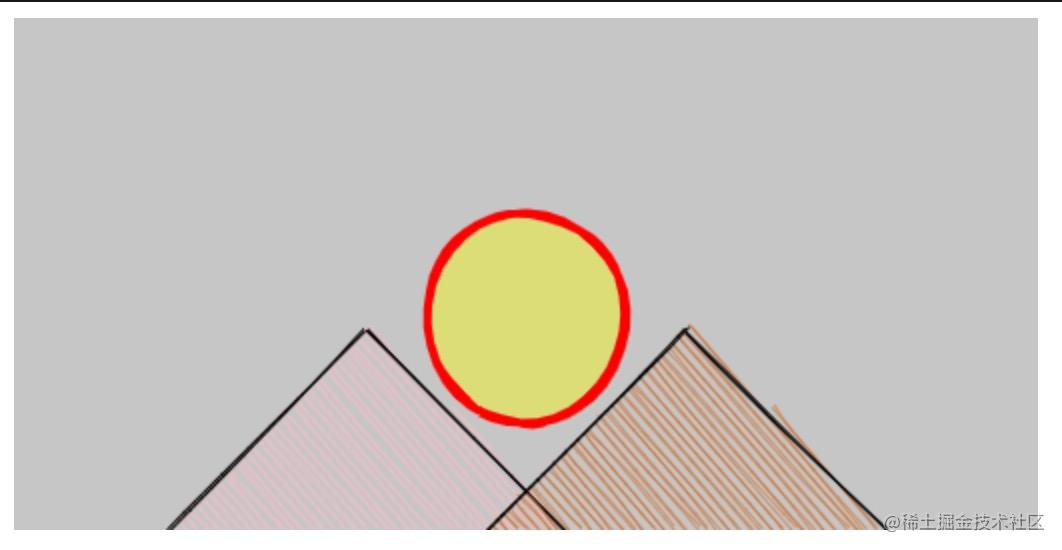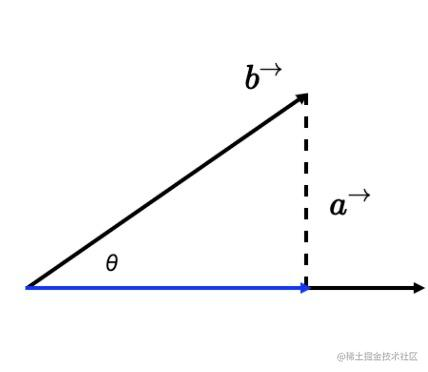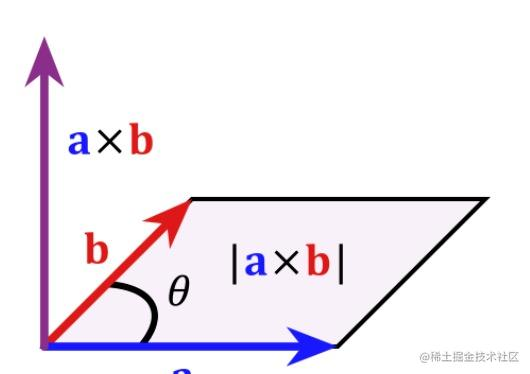# 一棵树-可视化之图形化基础之向量

• 可视化是前端可视化
• 图形是计算机图形学
• 向量就是那个向量，高中学过的，你懂的
• 树是那棵贼丑的树

## 过程

### 坐标系

Maaaaaaaaagic!

``````const canvas = document.querySelector('canvas')
const ctx = canvas.getContext('2d')
// 我们这里把原点定位在canvas左下角
ctx.translate(0, canvas.height)
// 关键步骤： 将canvasY轴方向翻转
ctx.scale(1, -1)

``````<canvas
width="512"
height="256"
style="display: block;margin: 0 auto;background-color: #ccc"
></canvas>

``````const canvas = document.querySelector('canvas')
const rc = rough.canvas(canvas)
rc.ctx.translate(0, canvas.height)
rc.ctx.scale(1, -1)

const cSun = [canvas.width / 2, 106]
const diameter = 100 // 直径

const hill1Points = {
start: [76, 0], // 起始点
top: [176, 100], // 顶点
end: [276, 0] // 终点
}

const hill2Points = {
start: [236, 0], // 起始点
top: [336, 100], // 顶点
end: [436, 0] // 终点
}

const hill1Options = {
roughness: 0.8,
stokeWidth: 2,
fill: 'pink'
}

const hill2Options = {
roughness: 0.8,
stokeWidth: 2,
fill: 'chocolate'
}

function createHillPath(point) {
const { start, top, end } = point

return `M\${start} \${start}L\${top} \${top}L\${end} \${end}`
}

function paint() {
rc.path(createHillPath(hill1Points), hill1Options)
rc.path(createHillPath(hill2Points), hill2Options)

rc.circle(cSun, cSun, diameter, {
stroke: 'red',
strokeWidth: 4,
fill: 'rgba(255, 255, 0, 0.4)',
fillStyle: 'solid'
})
}

paint()（是不是也能在画展上卖个不错的价格）

### 向量

#### 向量的方向

``````// 构造函数在本文稍后的地方介绍
const v = new Vector2D(1, 10)
const dir = Math.atan2(v.y, v.x)

#### 四则运算

##### 乘除va * vb = va.length * vb.length * cos(rad)va * vb = va.length * va.length * sin(rad)

#### 构造器

``````// 用一个长度为2的数组表示一个向量， 下标为0的位置表示x 下标为1的位置表示 y
class Vector2D extends Array {
constructor(x = 1, y = 0) {
super(x, y)
}

get x() {
return this
}

get y() {
return this
}

set x(v) {
this = v
}

set y(v) {
this = v
}

this.x = this.x + v.x
this.y = this.y + v.y
return this
}

length() {
return Math.hypot(this.x, this.y)
}

const [x, y] = this
this.x = x * c + y * -s
this.y = x * s + y * c
return this
}
}

### 动手画图

1. 准备一个 512 * 512 的画布
``````<html>
...
<canvas
width="512"
height="512"
style="display:block;margin:0 auto;background-color: #ccc"
></canvas>
...
</html>

1. 翻转 canvas 坐标系
``````const canvas = document.querySelector('canvas')
const ctx = canvas.getContext('2d')
ctx.translate(0, canvas.height)
ctx.scale(1, -1)

1. 定义绘制树枝的方法
``````/**
* 1. ctx canvas ctx 上下文对象
* 2. 起始向量
* 3. length 向量长度(树枝长度)
* 4. thickness 线段宽度
* 5. 单位向量 dir 旋转角度
* 6. bias 随机因子
*/
const canvas = document.querySelector('canvas')
const ctx = canvas.getContext('2d')
ctx.translate(0, canvas.height)
ctx.scale(1, -1)
ctx.lineCap = 'round'
console.log(canvas.width)
const v0 = new Vector2D(canvas.width / 2, 0)

function drawBranch(ctx, v0, length, thickness, rad, bias) {
ctx.beginPath()
ctx.lineWidth = thickness
ctx.moveTo(...v0)
ctx.lineTo(...v1)
ctx.stroke()
ctx.closePath()
}
// 定义好了之后我们先画一个树枝试试看
drawBranch(ctx, v0, 50, 10, Math.PI / 2, 1)

1. 递归画图
``````// 先定义收缩系数
const LENGTH_SHRINK = 0.9
const THICKNESS_SHRINK = 0.8
const BIAS_SHRINK = 1

function drawBranch(ctx, v0, length, thickness, rad, bias) {
//....

if (thickness > 2) {
// 画左树枝
const left =
Math.PI / 4 +
drawBranch(
ctx,
v1,
length * LENGTH_SHRINK,
thickness * THICKNESS_SHRINK,
left,
bias
)

// 画右树枝
const right = Math.PI / 4 + RAD_SHRINK * (rad - 0.2)
drawBranch(
ctx,
v1,
length * LENGTH_SHRINK,
thickness * THICKNESS_SHRINK,
right,
bias
)
}
}
drawBranch(ctx, v0, 50, 10, Math.PI / 2, 1)

``````function drawBranch(ctx, v0, length, thickness, rad, bias) {
// ...

if (thickness > 2) {
// 画左树枝
const left =
Math.PI / 4 + RAD_SHRINK * (rad + 0.2) + bias * (Math.random() - 0.5)
drawBranch(
ctx,
v1,
length * LENGTH_SHRINK,
thickness * THICKNESS_SHRINK,
left,
bias
)

// 画右树枝
const right =
Math.PI / 4 + RAD_SHRINK * (rad - 0.2) + bias * (Math.random() - 0.5)
drawBranch(
ctx,
v1,
length * LENGTH_SHRINK,
thickness * THICKNESS_SHRINK,
right,
bias
)
}
}
drawBranch(ctx, v0, 50, 10, Math.PI / 2, 1)

``````function drawBranch(ctx, v0, length, thickness, rad, bias) {
// ...

if (thickness < 5 && Math.random() < 0.3) {
const th = 6 + Math.random()

ctx.save()
ctx.strokeStyle = '#e4393c'
ctx.lineWidth = th
ctx.beginPath()
ctx.moveTo(...v1)
ctx.lineTo(v1.x, v1.y + 2)
ctx.stroke()
ctx.closePath()
ctx.restore()
}
}

// 这里增大了随机因子， 让树枝更加分散
drawBranch(ctx, v0, 50, 10, Math.PI / 2, 1)

## 总结

• 前夕
1天前
• 皮大大
23小时前•• yugasun
10天前••• 雅伊
18天前•• 效率君
14天前••• 三原
2月前